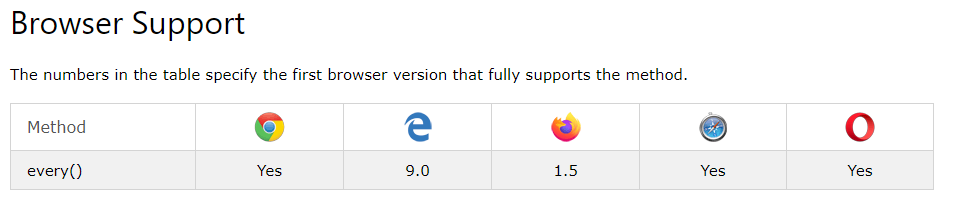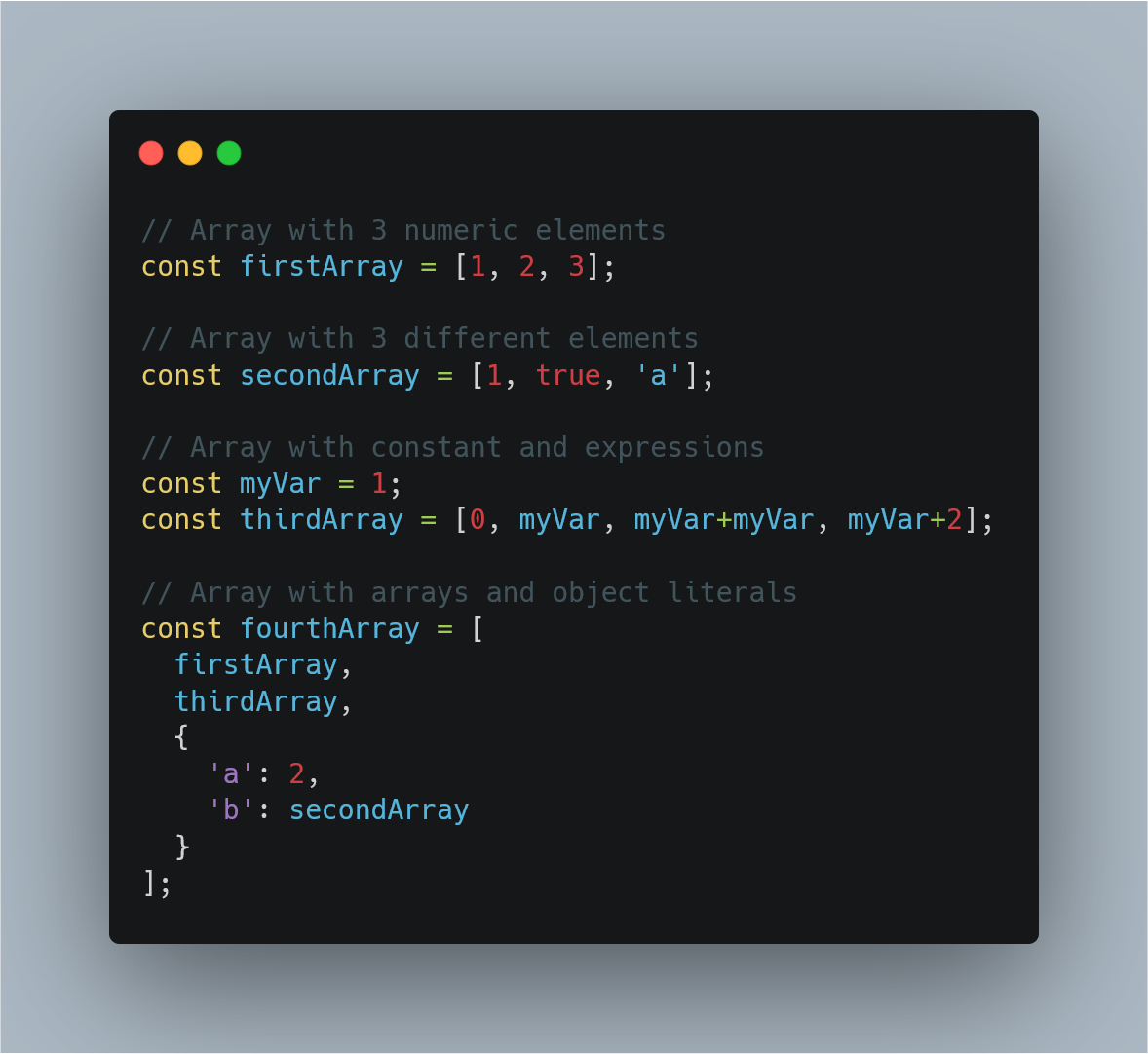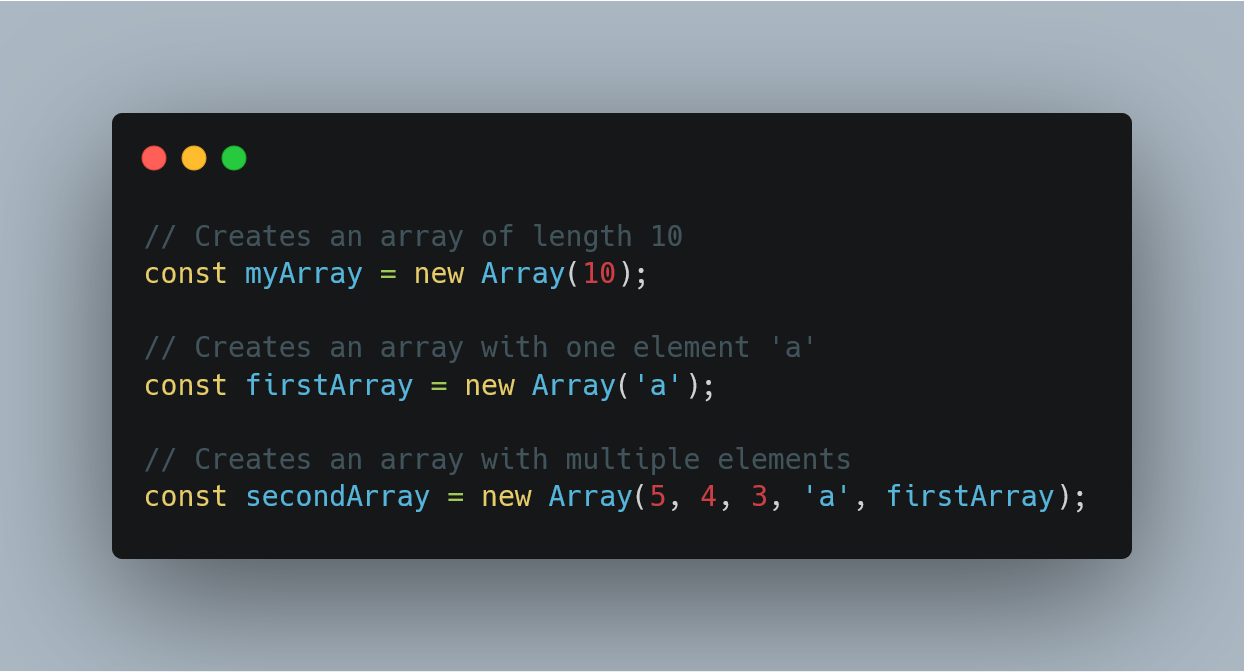1583550336

# How to Use .every() to Validate JavaScript Array Values

Arrays are a mainstay data structure; learning different and optimal ways to work with them is a critical skill for any programmer. Arrays have built-in methods intended to replace for loops.

The map(), filter(), and reduce() methods get most of the attention, but they’re not the only ones. If your task at hand is to validate all elements in an array, then you need to be using the every() method.

## The .every() Method

According to the MDN web docs, the `every()` method is called by passing a callback function. Each element in the arrow will be passed to the callback and if all elements return true, then `every()` will return true.

``````function isFive(n) {
return n == 5;
}

var nums = [5,5,5];

console.log(nums.every(isFive)); // true
``````

Our code example can be optimized in a few ways. If the callback will only be used once, an anonymous arrow function can be used in its place.

``````var nums = [5,5,5];

// full arrow syntax
console.log(nums.every((n) => { return n == 5; }));

// shorthand arrow syntax
console.log(nums.every(n => n == 5));
``````

If you’re working in an environment that supports ES6, you should be using let or const instead of var to define the array.

``````// use if nums will change
let nums = [5,5,5];

// use if nums will not change
const nums =  [5,5,5];
``````

## What About a for Loop?

If you’re clinging on to using Vanilla `for` loops, then let’s take a look at the previous example refactored as a `for` loop.

``````let isFive = true;
let nums = [5,5,5];

for(let i=0; i < nums.length; i++) {
if(nums[i] != 5) { isFive = false; }
}
``````

To me, the `for` loop is longer and harder to read. Using `every()` — regardless of what the actual callback is — lets the reader know that the goal is to validate all elements.

Using a `for…of` loop yields similar results.

``````let isFive = true;
let nums = [5,5,5];

for(n of nums) {
if(n != 5) { isFive = false; }
}
``````

Also, note that both of these examples require a negation, which further convolutes your code.

## Concerns With the every() Method

Unfortunately, `every()` is not perfect for all scenarios; there are some challenges with the method.The method also has fringe behaviors such as returning `true` when an empty array is passed.

``````let nums = [];

console.log(nums.every(n => n == 5)); // true
``````

This is because the callback is never invoked as there are no elements to pass to it. If you’d expect the empty array to return `false`, you can nest the callback within an `if` statement.

``````let nums = [];
let result;
if(nums.length) {
result = nums.every(n => n == 5);
} else {
result = false;
}
console.log(result); // false
``````

Since we’ve been doing some refactoring, let’s take another crack at optimizing the `if` statement with the ternary operator.

``````let nums = [];
let result = (nums.length) ? nums.every(n => n==5) : false;
``````

## Conclusion

Use the `every()` method to efficiently and semantically validate your array elements.

If this is new to you, what are some blocks you plan on refactoring? If you’re a seasoned user, what are your favorite use cases? Share your experiences below and thanks for reading!

#javascript #programming

## Buddha Community1624388400

## JavaScript Arrays Tutorial. DO NOT MISS!!!

Learn JavaScript Arrays

📺 The video in this post was made by Programming with Mosh
The origin of the article: https://www.youtube.com/watch?v=oigfaZ5ApsM&list=PLTjRvDozrdlxEIuOBZkMAK5uiqp8rHUax&index=4
🔥 If you’re a beginner. I believe the article below will be useful to you ☞ What You Should Know Before Investing in Cryptocurrency - For Beginner
⭐ ⭐ ⭐The project is of interest to the community. Join to Get free ‘GEEK coin’ (GEEKCASH coin)!
Thanks for visiting and watching! Please don’t forget to leave a like, comment and share!

#arrays #javascript #javascript arrays #javascript arrays tutorial1602154740

## Fill and Filter in Array in JavaScript

By the word Array methods, I mean the inbuilt array functions, which might be helpful for us in so many ways. So why not just explore and make use of them, to boost our productivity.

Let’s see them together one by one with some amazing examples.

### Array.fill():

The `_fill()_` method changes all elements in an array to a static value, from a start index (default `_0_`) to an end index (default `_array.length_`). It returns the modified array.

In simple words, it’s gonna fill the elements of the array with whatever sets of params, you pass in it. Mostly we pass three params, each param stands with some meaning. The first param value: what value you want to fill, second value: start range of index(inclusive), and third value: end range of index(exclusive). Imagine you are going to apply this method on some date, so that how its gonna look like eg: array.fill(‘Some date’, start date, end date).

``````NOTE: Start range is inclusive and end range is exclusive.
``````

Let’s understand this in the below example-

``````//declare array
var testArray = [2,4,6,8,10,12,14];

console.log(testArray.fill("A"));
``````

When you run this code, you gonna see all the elements of `testArray` will be replaced by `'A'` like `[“A”,"A","A","A","A","A","A"]`.

#javascript-tips #array-methods #javascript-development #javascript #arrays1606912089

## How to create a calculator using javascript - Pure JS tutorials |Web Tutorials

### In this video I will tell you How to create a calculator using javascript very easily.

#how to build a simple calculator in javascript #how to create simple calculator using javascript #javascript calculator tutorial #javascript birthday calculator #calculator using javascript and html1590478483

## Convert String To Array Using Javascript Split Method

The easiest approach to use javascript built-in method String.split().

#javascript #javascript string #string to array #morioh #array1597470780

## Introduction

Arrays are a structure common to all programming languages so knowing what they are and having a firm grasp on what you’re able to accomplish with Arrays will take you a long way in your journey as a software developer. The code examples I share in this post will be in JavaScript but the concepts are common among all languages. What you learn here can easily be translated to any other language you work with.

In this post I’ll be covering how to perform the create, read update and delete operations using arrays, some common functions that come with the Array prototype and also how to implement them.

## What is an Array

Before we jump into the juicy bits of Arrays, lets quickly gloss over what they are. Arrays

• are a fundamental data type in JavaScript
• are an ordered collection of values called **elements **that are stored at and accessed via an index
• are untyped, meaning that the elements of an array could be of different types. This allows us to create complex arrays such as an array of objects or even an array of arrays (multidimensional arrays)
• can have elements that are constants or expressions
• have a property called length that tells you the number of elements in the array
• inherit properties from `Array.prototype` that includes a wide variety useful functions that can be called from arrays or `array-like` objects

## CRUD operations using Arrays

If you’re not familiar with the term CRUD it stands for Create, Read, Update and Delete. In this section we’ll go through each one of these operations and cover different ways you can perform each one.

### Creating Arrays

There are several ways you can create an Array but the most common ways are by using

• the Array literal syntax
• the Array constructor i.e. `new Array()`

Lets take a look at each one with examples

### Array literal

The array literal is the most common way of creating an array. It uses the square brackets as a notion of a container followed by comma separated values inside the square brackets. The following examples show how to use the array literal syntax and how arrays are untyped i.e. can contain elements of different types.Examples of untyped arrays in JavaScript created with the array literal syntax.

### Array constructor

Another way to create an array is through the Array constructor.

``````const myArray = new Array();
``````

Using the Array constructor, as shown above, is the same as creating an array with the array literal syntax. i.e.

``````// The following two lines behave exactly the same way i.e. both create an empty arrays

const myArray = new Array();
const myOtherArray = [];
``````

The array constructor, however, is able to receive arguments that allow it to behave in different ways depending on the number and type of arguments passed to it.

• You can pass a single numeric argument which creates an array of the specified length. This option is mostly used when you know how many elements you’ll be placing in the array
``````const myArray = new Array(5);
``````

Note: If you want to define the array with a specified size, as shown above, the argument passed must be a numeric value. Any other type would be considered as the first element that’ll be placed in the array.

• Or you can pass two or more arguments or a non-numeric argument to place the values inside the array. This works the same way as shown in the array literal examples.Examples of arrays created by using the Array constructor in JavaScript

As stated earlier, these two ways are the most common ways of creating arrays that you’ll see and use 99% of the time. There are a few other ways but we won’t dive deep into how they work. They are

• the spread operator `const someArray = […someOtherArray]`
• the static method `Array.of()`
• and the static method `Array.from()`

#javascript #web-development #javascript-tips #javascript-development #javascript-arrays #sql